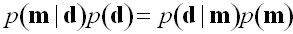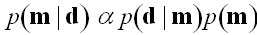Sampling Methods to Determine Optimal Models, Model Resolution and Model Choice in Earth Science Modelling Problems

Kerry Gallagher1, Soren Nielsen2, Karl Charvin3, John Stephenson3, Malcolm Sambridge4, and Chris Holmes5
1Géosciences Rennes, University of Rennes, France
2Dept of Earth Sciences, University of Aarhus, Denmark
3Dept of Earth Sciences and Engineering, Imperial College London, England
4Research School of Earth Sciences, Australian National University, Australia
5Dept. of Statistics, University of Oxford, England

Characterisation of uncertainty is an integral part of a modelling workflow and a variety of different methodologies are available. In general, the preferred methods involve some form of inversion approach, which incorporates finding a best model (optimisation) and an assessment of the resolution of such a model (appraisal). Methods which are efficient in the former aspect are not always useful for the latter, and vice-versa. Many optimisation methods, for example, often rely on some form of linearising approximation and the assumption of Gaussian statistics to address uncertainty. Here, we present an overview of sampling based methods which avoid such limitations, and focus in particular on Markov chain Monte Carlo (MCMC) approaches formulated in a Bayesian framework.

In this approach, we define a prior probability distribution on the unknown model parameters, p(m), i.e. what we think is quantitatively reasonable for our particular geological setting, in the absence of any observed data, d. Then, Bayes’ rule expresses the relationship between the data and the model as(1)

where the terms p(d|m), and p(m|d) are the likelihood (or data fit) and the posterior probability (of the model given the data). The term, p(d), is often referred to as the evidence and is equivalent to the righthand side integrated over all possible models. In many situations, this can be regarded as constant, so we can write (1) as a proportionality relationship(2)

Thus, the posterior distribution can be thought of how our prior knowledge of the model parameters is updated once we have some observed data. Clearly, if the prior and the posterior distributions are the same, we have learnt nothing from the data. In general, this is not the case, and the posterior distribution provides a full characterisation of the uncertainty in the model.

Markov chain Monte Carlo exploits (2) through an iterative sampling strategy based on a theoretically derived acceptance-rejection criterion, in which the current model is compared to a proposed model (determined by perturbing the current model). In a simple case the proposed model is accepted always if it fits the data better than the current model, but if not it is may be accepted on a probabilistic basis. In this way, it is possible to sample the posterior distribution without having to know the value of p(d). This strategy has been applied to thermal history modelling in 3D to address the uncertainty inherent in calibration of heat flow to thermal indicators such as vitrinite reflectance.

Recent developments in MCMC allow for variable dimension problems to be addressed, such that we do not need to specify the number of unknown parameters a priori. Rather, the trans-dimensional form of MCMC, known as Reversible Jump (MCMC), has the number of model parameters as an unknown which can be estimated. This is most natural for problems which have an obviously discrete number of parameters, and has been applied for estimating the number of components in a mixture of detrital mineral ages in a sediment. It is also a practical strategy to choose between models of varying complexity, such as models with a similar structure but differing numbers of parameters, and has been applied in the context of the inference of sea-level and sediment flux from stratigraphic architecture. Finally, the fixed and trans-dimensional methods can be combined to allow multiple data sets to be grouped together, providing better resolution of uncertainty in model inference. The trans-dimensional aspect here is to determine the appropriate groupings, while simultaneously fitting an unknown function to each data group. This has been applied to thermochronological data in 3D, where each data group is inferred to belong to a particular structural domain and all samples in a given group have experienced a common cooling history.

We shall present an overview of these methods, with a particular emphasis on their application to the Earth Science problems mentioned above.

AAPG Search and Discover Article #90066©2007 AAPG Hedberg Conference, The Hague, The Netherlands